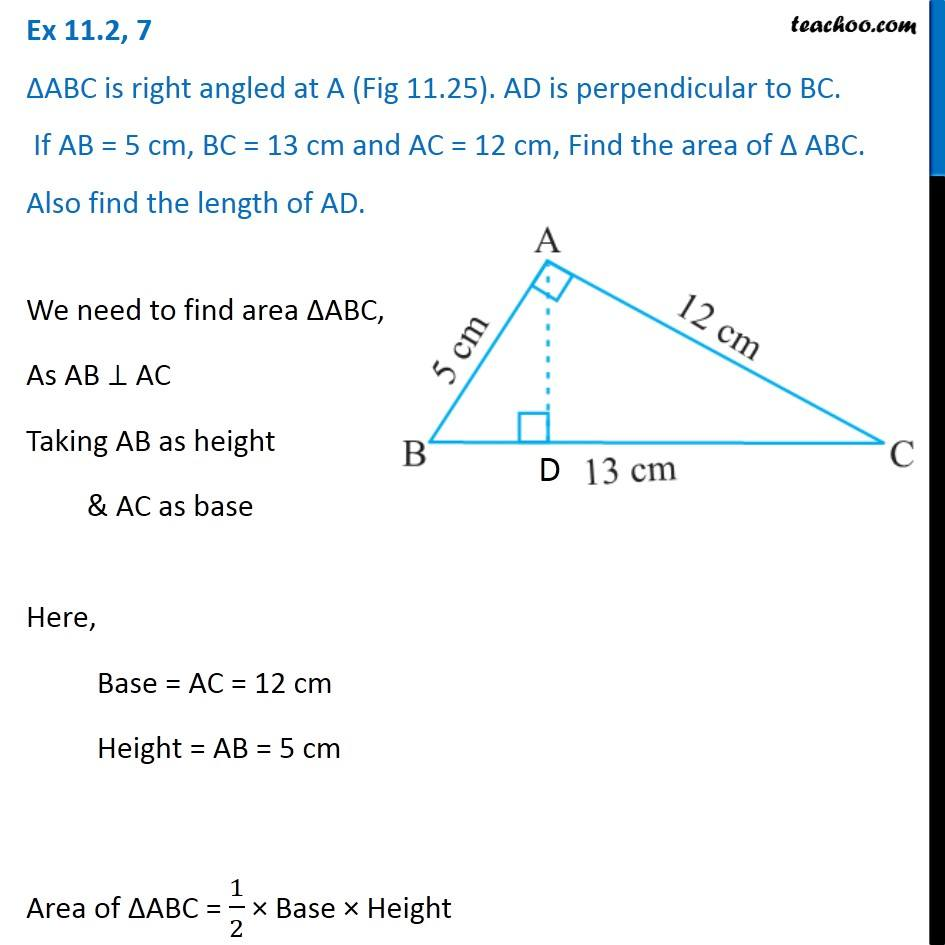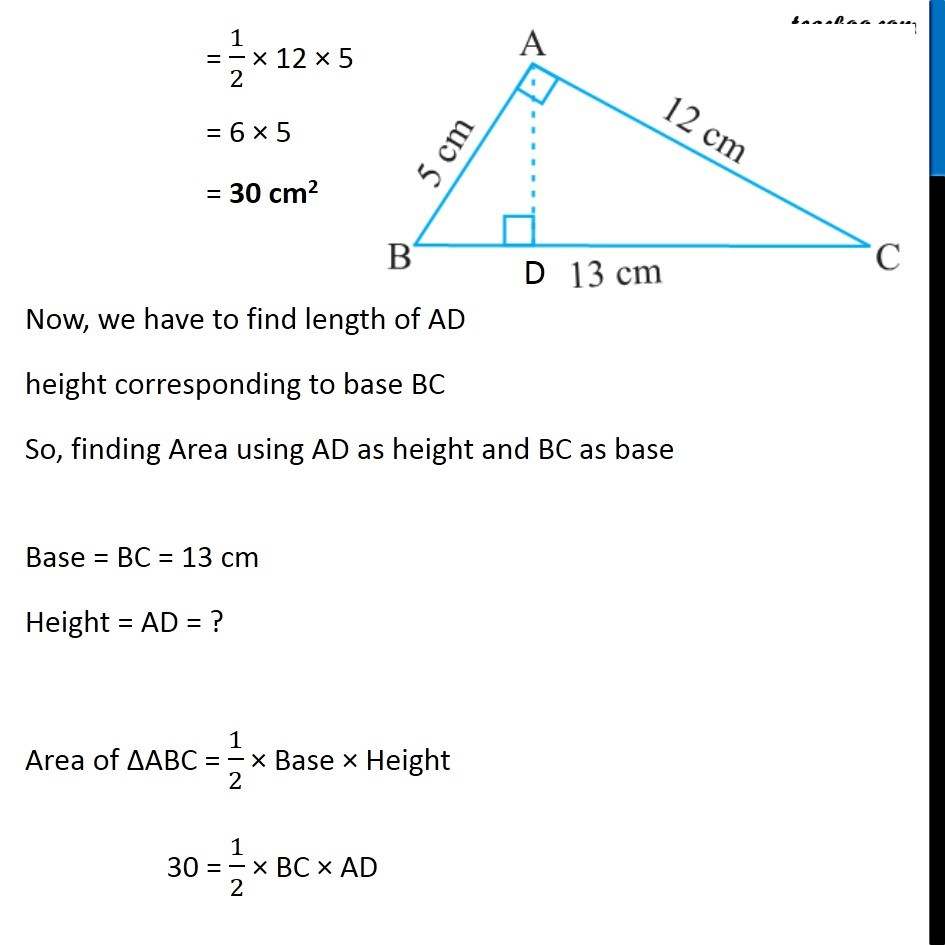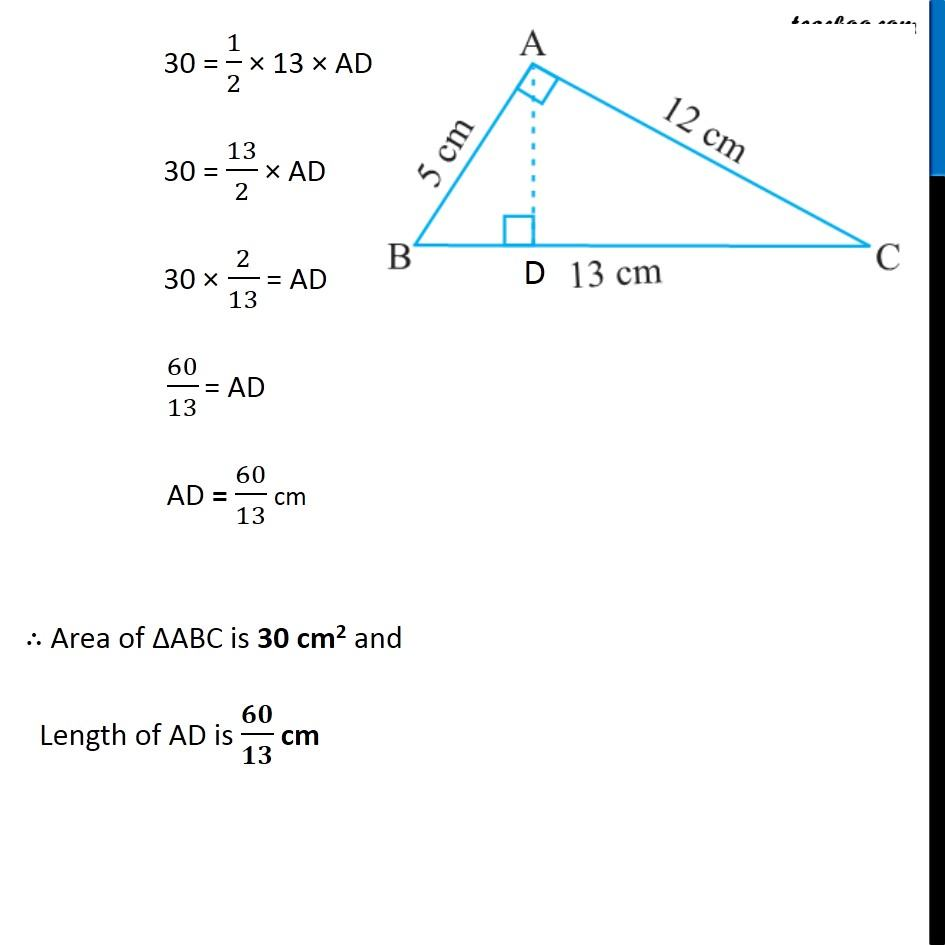1. Chapter 11 Class 7 Perimeter and Area
2. Serial order wise
3. Ex 11.2

Transcript

Ex 11.2, 7 ΔABC is right angled at A (Fig 11.25). AD is perpendicular to BC. If AB = 5 cm, BC = 13 cm and AC = 12 cm, Find the area of Δ ABC. Also find the length of AD. We need to find area ∆ABC, As AB ⊥ AC Taking AB as height & AC as base Here, Base = AC = 12 cm Height = AB = 5 cm Area of ∆ABC = 1/2 × Base × Height = 1/2 × 12 × 5 = 6 × 5 = 30 cm2 Now, we have to find length of AD height corresponding to base BC So, finding Area using AD as height and BC as base Base = BC = 13 cm Height = AD = ? Area of ∆ABC = 1/2 × Base × Height 30 = 1/2 × BC × AD 30 = 1/2 × 13 × AD 30 = 13/2 × AD 30 × 2/13 = AD 60/13 = AD AD = 60/13 cm ∴ Area of ∆ABC is 30 cm2 and Length of AD is 𝟔𝟎/𝟏𝟑 cm

Ex 11.2